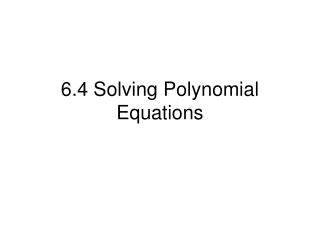DownloadDownload Presentation6.4 Solving Polynomial Equations

# 6.4 Solving Polynomial Equations

Download Presentation## 6.4 Solving Polynomial Equations

- - - - - - - - - - - - - - - - - - - - - - - - - - - E N D - - - - - - - - - - - - - - - - - - - - - - - - - - -
##### Presentation Transcript

1. 6.4 Solving Polynomial Equations

2. One of the topics in this section is finding the cube or cube root of a number. • A cubed number is the solution when a number is multiplied by itself three times. • A cube root “undos” the cubing operation just like a square root would.

3. Calculator Function – How to take the cube root of a number • To take the cube root of a number, press MATH, then select option 4. Example: What is ? 24

4. Solving Polynomials by Graphing • We start getting into more interesting equations now . . . Ex: x3 + 3x2 = x + 3 Problem: Solve the equation above, using a graphing calculator

5. Solving Polynomials by Graphing • What about something like this??? Ex: x3 + 3x2 + x = 10 Use the same principal; plug the first part of the equation in for Y1; the solution (10) for Y2; then find the intersection of the two graphs.

6. FACTORING AND ROOTSCUBIC FACTORING Difference of Cubes a³ - b³ = (a - b)(a² + ab + b²) Sum of Cubes a³ + b³ = (a + b)(a² - ab + b²) Question: if we are solving for x, how many possible answers can we expect? 3 because it is a cubic!

7. CUBIC FACTORINGEX- factor and solve 8x³ - 27 = 0 a³ - b³ = (a - b)(a² + ab + b²) 8x³ - 27 = (2x - 3)((2x)² + (2x)3 + 3²) (2x - 3)(4x² + 6x + 9)=0 X= 3/2 Quadratic Formula

8. CUBIC FACTORINGEX- factor and solve x³ + 343 = 0 a³ + b³ = (a + b)(a² - ab + b²) x³ + 343 = (x + 7)(x² - 7x + 7²) (x + 7)(x² - 7x + 49)=0 X= -7 Quadratic Formula

9. Let’s try one • Factor a) x3-8 b) x3-125

10. Let’s try one • Factor a) x3-8 b) x3-125

11. Let’s Try One • 81x3-192=0 Hint: IS there a GCF???

12. Let’s Try One • 81x3-192=0

13. Factor by Using a Quadratic Form Ex: x4-2x2-8 = (x2)2 – 2(x2) – 8 Substitute a in for x2 = a2 – 2a – 8 This is something that we can factor (a-4)(a+2) Now, substitute x2 back in for a (x2-4)(x2+2) (x2-4) can factor, so we rewrite it as (x-2)(x+2) Since this equation has the form of a quadratic expression, we can factor it like one. We will make temporary substitutions for the variables So, x4-2x2-8 will factor to (x-2)(x+2)(x2+2)

14. Let’s Try One • Factor x4+7x2+6

15. Let’s Try One • Factor x4+7x2+6